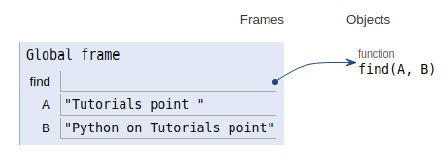# Python program to find uncommon words from two Strings

Problem statement − We are given two strings, we need to get the uncommon words from the given strings.

Now let’s observe the solution in the implementation below −

## Example

Live Demo

# uncommon words
def find(A, B):
# count
count = {}
# insert in A
for word in A.split():
count[word] = count.get(word, 0) + 1
# insert in B
for word in B.split():
count[word] = count.get(word, 0) + 1
# return ans
return [word for word in count if count[word] == 1]
# main
A = "Tutorials point "
B = "Python on Tutorials point"
print("The uncommon words in strings are:",find(A, B))

## Output

The uncommon words in strings are: ['Python', 'on']All the variables are declared in the local scope and their references are seen in the figure above.

## Conclusion

In this article, we have learned about how we can make a Python program to find uncommon words from two Strings